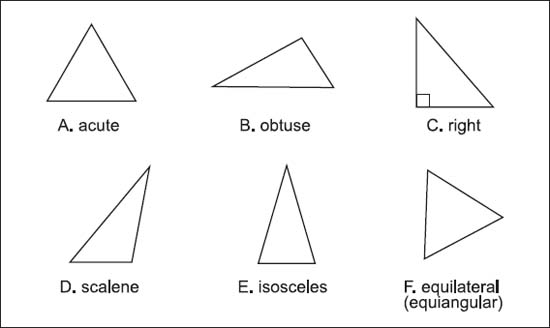NextPrevious

# What are the parts of right and isosceles triangles?

There are special names for the parts of a right triangle. The hypotenuse is the side opposite the 90 degree angle—the side that will always be the triangle’s longest. The shorter sides are called the legs.

Similar to the right triangle, the isosceles triangle has specific names for its angles and sides: Congruent (equal) sides are, as with the right triangle, called the legs; the angle formed by these two legs is called the vertex angle; and the base is the side opposite the vertex angle. The two angles that are formed by the base and the legs are called the triangle’s base angles.The basic types of triangles are acute (A), obtuse (B), right (C), scalene (D), isosceles (E), and equilateral (F).

Close

This is a web preview of the "The Handy Math Answer Book" app. Many features only work on your mobile device. If you like what you see, we hope you will consider buying. Get the App